# Texas Go Math Grade 1 Lesson 10.4 Answer Key Skip Count by Fives

Refer to our Texas Go Math Grade 1 Answer Key Pdf to score good marks in the exams. Test yourself by practicing the problems from Texas Go Math Grade 1 Lesson 10.4 Answer Key Skip Count by Fives.

## Texas Go Math Grade 1 Lesson 10.4 Answer Key Skip Count by Fives

Explore

Skip count by fives. Write to show how many fingers.Skip Counting Up in Fives. To skip count by 5, add five to get to the next number. Keep adding 5 to each number to get to the next and do not count the numbers in between. We start at zero and continue to add five to get to the next numbers. When skip counting in fives from zero, the first few numbers are simply the numbers in the five times table.FOR THE TEACHER • Read the following problem. How many hands would it take to show 50 fingers?
In the above problem, there are 10 hands. In each hand, there are 5 fingers. So for 10 hands, 10*5=50 fingers are there.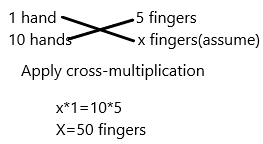Math Talk
Mathematical Processes

Explain When is it easier to count a set of objects by fives?
We count by fives quite often; counting money and telling the time with one example. The picture below shows how to count forward by fives starting from 30.Model and Draw

Skip count the candles by fives to find how many.
How many are you counting each time?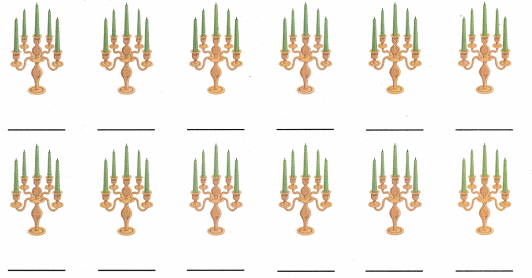1. To skip count in fives, keep adding 5 to get the next number.
2. We do not count the numbers in between.
3. We will count up in fives from 5.
4. We start at 5 and continue to add 5 to get the next numbers.
5. When we skip counting from 5s, the numbers are in the five times table.
So we have: 5, 10, 15, 20, 25, 30, 35, 40, 45, 50, 55, 60.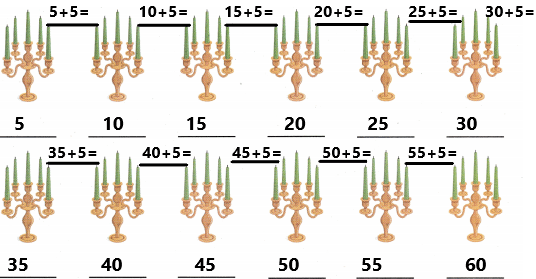Share and Show

Skip count. Count the petals on the flowers by fives. Write how many.

Think: What is the best way to count these objects.

Question 1.1. To skip count in fives, keep adding 5 to get the next number.
2. We do not count the numbers in between.
3. We will count up in fives from 5.
4. We start at 5 and continue to add 5 to get the next numbers.
5. When we skip counting from 5s, the numbers are in the five times table.
So we have: 5, 10, 15, 20, 25, 30, 35, 40, 45, 50, 55, 60, 65, 70, 75, 80, 85, 90, 95, 100.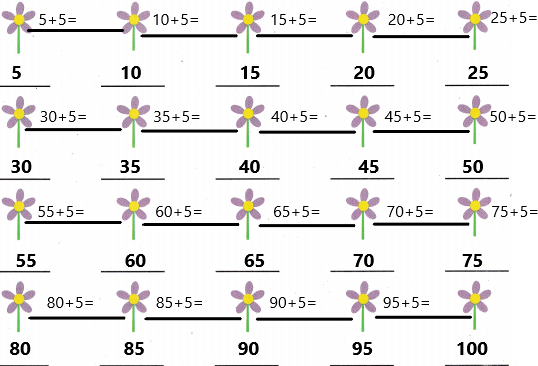Problem Solving

Skip count. Write how many.

Question 2.____________ balls
Explanation:
1. To skip count in fives, keep adding 5 to get the next number.
2. We do not count the numbers in between.
3. We will count up in fives from 5.
4. We start at 5 and continue to add 5 to get the next numbers.
5. When we skip counting from 5s, the numbers are in the five times table.
So we have: 5, 10, 15, 20, 25, 30, 35, 40, 45, 50, 55, 60, 65, 70, 75.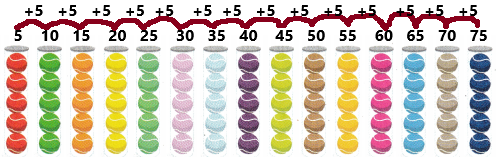Question 3._________ points
Explanation:
1. To skip count in fives, keep adding 5 to get the next number.
2. We do not count the numbers in between.
3. We will count up in fives from 5.
4. We start at 5 and continue to add 5 to get the next numbers.
5. When we skip counting from 5s, the numbers are in the five times table.
So we have: 5, 10, 15, 20, 25, 30, 35, 40, 45, 50, 55, 60, 65.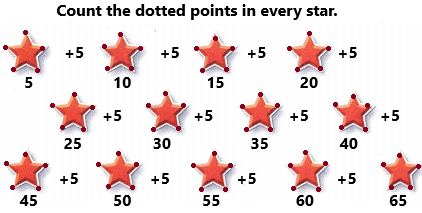Use skip counting by fives to solve. Write or draw to explain.

Question 4.
H.O.T. There are five arms on each starfish. How many arms are on 22 starfish?__________ arms
Explanation:
1. To skip count in fives, keep adding 5 to get the next number.
2. We do not count the numbers in between.
3. We will count up in fives from 5.
4. We start at 5 and continue to add 5 to get the next numbers.
5. When we skip counting from 5s, the numbers are in the five times table.
So we have: 5, 10, 15, 20, 25, 30, 35, 40, 45, 50, 55, 60, 65, 70, 75, 80, 85, 90, 95, 100, 105, 110.
The number of arms does starfish have=5
The total number of arms does 22 starfish =X
We need to find out that.
For 1 starfish, 5 arms. So for 22 starfish, the number of arms will be how many.Question 5.
H.O.T. Multi-Step Emma saves 5 cents a day. How many cents does she have in one week?
__________ cents
One week has 7 days.
Here Emma saves 5 cents every day.
The cents she saves in a week=7*5=35.
Therefore, she saves 35 cents a week.

Question 6.
Analyze Jake’s farm has 10 ponds. Each pond has 5 fish. How many fish are on Jake’s farm? Skip count by fives.
(A) 20
(B) 50
(C) 100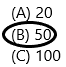Explanation:
The total number of ponds Jakes has=10
The number of fishes in each pond=5
The total number of fishes in 10 ponds=X
For 1 pond, 5 fish. Then for 10 ponds, the number of fishes need to be found. Apply cross-multiplication.Question 7.
Gary likes to count his puzzle pieces by fives. Which numbers complete the skip count by fives sequence?
95, _______, 105, 110, _______
(A) 100 and 115
(B) 96 and 111
(C) 100 and 111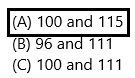Explanation:
1. To skip count in fives, keep adding 5 to get the next number.
2. We do not count the numbers in between.
3. We will count up in fives from 5.
4. We start at 95 and continue to add 5 to get the next numbers.
5. When we skip counting from 5s, the numbers are in the five times table.
So we have: 95, 100, 105, 110, 115.

Question 8.
Multi-Step Jenna collects baseball cards. She groups them in fives, and puts 2 groups in 1 stack. Jenna has 10 stacks of cards. How many cards does Jenna have?
(A) 50
(B) 100
(C) 25Explanation:
The number of baseball cards in each group=5
The number of groups in each stack=2
The total number of cards in each stack=5*2=10
The total number of stacks=10
The total number of cards in 10 stacks=X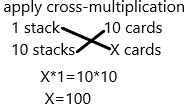Question 9.
Texas Test Prep Which number comes after 95 when skip counting by fives?
(A) 96
(B) 105
(C) 100Explanation:
Add 5 to get the next number.
I start at 95, so add 5 to the 95.
95+5=100.

TAKE HOME ACTIVITY • Have your child use buttons or beans to make groups of five. Then have him or her count by fives to tell how many objects.
Answer: Give buttons to the child and make them count by using skip count using by 5s technique.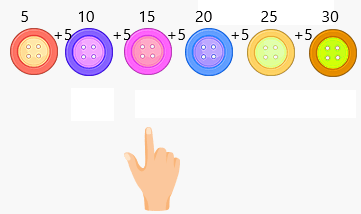### Texas Go Math Grade 1 Lesson 10.4 Homework and Practice Answer Key

Skip count. Count the fish in the bowls by fives. Write how many.

Question 1.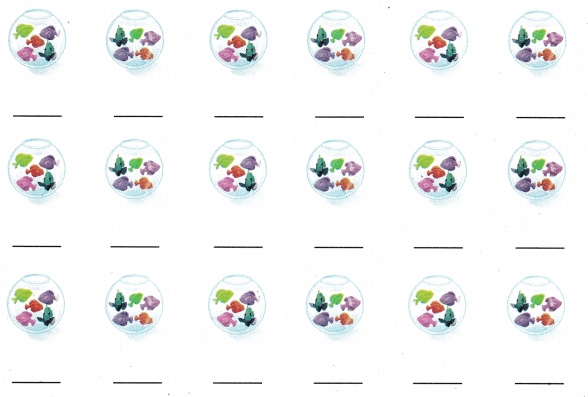Explanation:
1. To skip count in fives, keep adding 5 to get the next number.
2. We do not count the numbers in between.
3. We will count up in fives from 5.
4. We start at 5 and continue to add 5 to get the next numbers.
5. When we skip counting from 5s, the numbers are in the five times table.
So we have: 5, 10, 15, 20, 25, 30, 35, 40, 45, 50, 55, 60, 65, 70, 75, 80, 85, 90.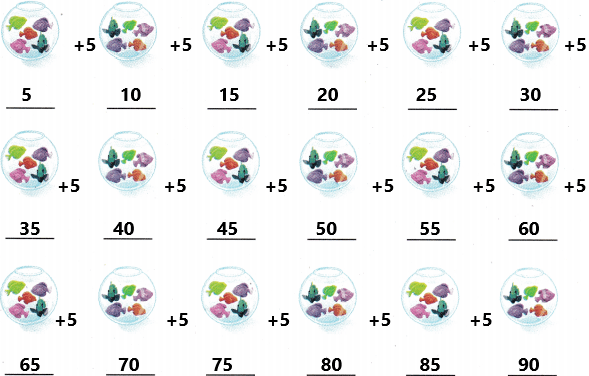Problem Solving

Use skip counting by fives to solve. Write or draw to explain.

Question 2.
Chet counts balloons for a party. He counts 13 bunches of balloons. Each bunch has 5 balloons. How many balloons does Chet count?
__________ balloons
Explanation:
The number of bunches of balloons he counts=13.
The number of balloons has in each bunch=5
The total number of balloons he count=XLesson Check

Question 3.
Willy makes groups of 5 to count his crayons. He makes 9 groups. How many crayons does Willy have?(A) 90
(B) 45
(C) 18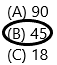Explanation:
The number of crayons has in each group=5
The number of groups=9
The total number of crayons in 9 groups=5*9=45.

Question 4.
Count by fives. Which numbers are not shown?
90, 95, _______, 105, _______, 115
(A) 96 and 106
(B) 100 and 106
(C) 100 and 110Explanation:
1. To skip count in fives, keep adding 5 to get the next number.
2. We do not count the numbers in between.
3. We will count up in fives from 5.
4. We start at 90 and continue to add 5 to get the next numbers.
5. When we skip counting from 5s, the numbers are in the five times table.
So we have: 90, 95, 100, 105, 110,115.

Question 5.
Multi-Step There are 5 chickens in each pen. There are 4 pens in the barn. There are 2 pens outside. How many chickens are there?(A) 30
(B) 40
(C) 20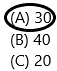Explanation:
The number of chickens in each pen=5
The total number of pens=4+2=6.
The total number of chickens in 6 pens=5*6=30.

Question 6.
Write the number that comes after 100 when skip counting by fives.